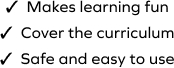# Regroup to Subtract 2-Digit From 4-Digit Number

SplashLearn - The complete PreK - Grade 5 Math & ELA Learning Program Built for Your Child
Home > Regroup to Subtract 2-Digit From 4-Digit Number
The game involves working with a set of problems on the column method to find the difference, which helps young learners solidify their understanding of the concept. The student will regroup to subtract 2-digit from 4-digit numbers in this game. Students will choose the correct answer from the given options to solve the problems.